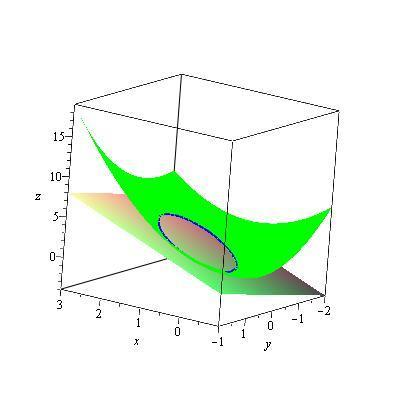# Curve of intersection of a plane and function

• MechanicalBrank
You need to figure out what the original function is, and then you can use the equations to find z. From what you've said, z is the derivative of f(x, y).

## Homework Statement

f(x,y) = x^2 + xy + y^2 and z=2x+y intersect, find a parameterization of the curve where they intersect.

## The Attempt at a Solution

I am lost. I know that z is the partial derivative of the original function, if that's of any use. I can visualize it but not quite sure how to begin calculating.

Last edited:
MechanicalBrank said:

## Homework Statement

f(x,y) = x^2 + xy + y^2 and z=2x+y intersect, find a parameterization of the curve where they intersect.

## The Attempt at a Solution

I am lost. I know that z is the partial derivative of the original function, if that's of any use.
It would help to know what is the original function, as well as the actual problem you're working on. From the equations above, f(x, y) is a z value, so you could equate x2 + xy + y2 with 2x + y. I'm not sure this leads to anything informative, so I'm wondering if you made a mistake somewhere.
MechanicalBrank said:
I can visualize it but not quite sure how to begin calculating.

MechanicalBrank said:

## Homework Statement

f(x,y) = x^2 + xy + y^2 and z=2x+y intersect, find a parameterization of the curve where they intersect.

## The Attempt at a Solution

I am lost. I know that z is the partial derivative of the original function, if that's of any use. I can visualize it but not quite sure how to begin calculating.

You seem to know something that is wrong: z is NOT the partial derivative of the original function (whatever that function is, since you do not say).

MechanicalBrank said:
not quite sure how to begin calculating.

A brute force approach is to let the parameter be $t$. Set $x = t$. Substitute for $t$ for $x$ in the equation $x^2 + xy + y^2 = 2x + y$. Solve for $y$ in terms of $t$.

There might be a clever way. Solving a quadratic equation for y whose coefficients are expressions in t isn't very elegant.

Consider the surface $z = x^2 + xy + y^2 - (2x + y)$. The requested intersection is a level curve of this surface for $z = 0$. Do you know any slick ways to parameterize the level curves of a surface? ( I haven't solved the problem myself, so I'm just speculating.) The value of the directional derivative of the surface along tangents to the level curve should be zero. Perhaps that's a useful fact.

Let f(x,y)=x^2 + xy + y^2 for all x in R2.
Find a parameterization of the curve given by the intersection of f(x,y) and the plane z=2x+y. That's the problem exactly as on paper.

MechanicalBrank said:
Let f(x,y)=x^2 + xy + y^2 for all x in R2.
Find a parameterization of the curve given by the intersection of f(x,y) and the plane z=2x+y. That's the problem exactly as on paper.

It should have said "find a parametrization of the curve of intersection of the two surfaces ##z = f(x,y) ## and ##z = 2x + y##."

I think I solved it.

In case anyone is still interested, I think the "natural" way to parameterize that intersection is to take the equation$$x^2+xy + y^2 - 2x -y = 0$$which is the projection of the intersection curve in the xy plane. Rotate and translate that to get its center and the standard form of the equation of the ellipse$$\frac {(x-x_0)^2}{a^2} + \frac {(y-y_0)^2}{b^2} = 1$$. Then let $$x =x_0+a\cos t,~~y = y_0 + b\sin t, z = 2x+y$$With a bit of help from Maple you get$$\vec r(t) = \langle 1 +\frac 1 {\sqrt 3} \cos t -\sin t,\frac 1 {\sqrt 3} \cos t +\sin t,2 + \sqrt 3\cos t -\sin t\rangle$$
Here's a picture:•MechanicalBrank
MechanicalBrank said:

## Homework Statement

f(x,y) = x^2 + xy + y^2 and z=2x+y intersect, find a parameterization of the curve where they intersect.

## Homework Equations

I presume you mean the intersection or z= x^2+ xy+ y^2 and z= 2x+ y. That intersection is given by x^2+ xy+ y^2- 2x- y= 0. It is possible, by rotating the axes, to remove the "xy" term getting an equation of an ellipse or other conic section for which it is easy to find parameters. Then use z= 2x+ y to get a parametric equation for z.

3. The Attempt at a Solution
I am lost. I know that z is the partial derivative of the original function, if that's of any use. I can visualize it but not quite sure how to begin calculating.

•MechanicalBrank